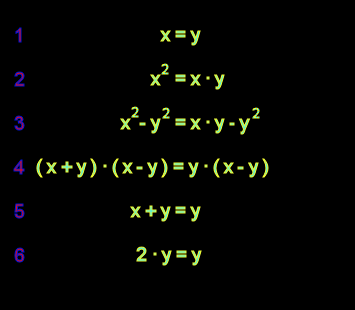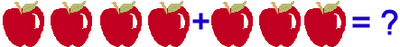## 2006-12-13

### A small math problemA minor exercise, if you will. Since nobody could solve the extremely annoying integral problem I gave last month, we'll try this one where I know the answer and can simply laugh when others don't get this.

In step (1) we declare x=y for all values of x. In step (2) both sides are multiplied by x. In step (3) y-squared is subtracted from both sides. In step (4) the left side is factored. In step (5) the x-y term is divided out on both sides, and in step (6) we use the equation (1) to replace the x with y, showing the 2y=1y for all y (and therefore proving that 2=1).

This is obviously bunk. The question is, where did we screw up? Those mathematicall inclined are welcome to respond in. Those who aren't can do the following problem instead:Nathan Giesbrecht said...

In step 5 when you divide both sides by (x-y) you are, in fact, dividing by zero.

Now maybe it's time for me to look at that integral...

Nathan Giesbrecht said...

My stab at the integral:

2/3(Ax^5/5 - Bx^4/4 + Cx^3/3 -Dx^2/2)^3/2

I know its wrong, and virtually unreadable, but I'd like to know the answer!